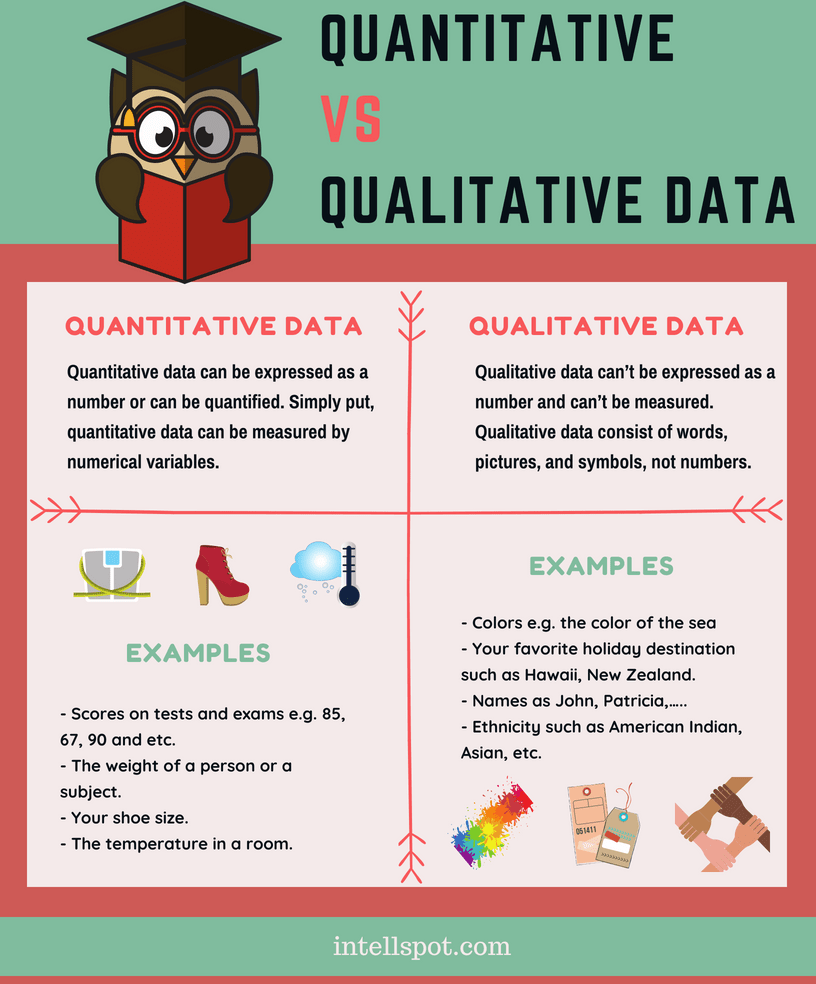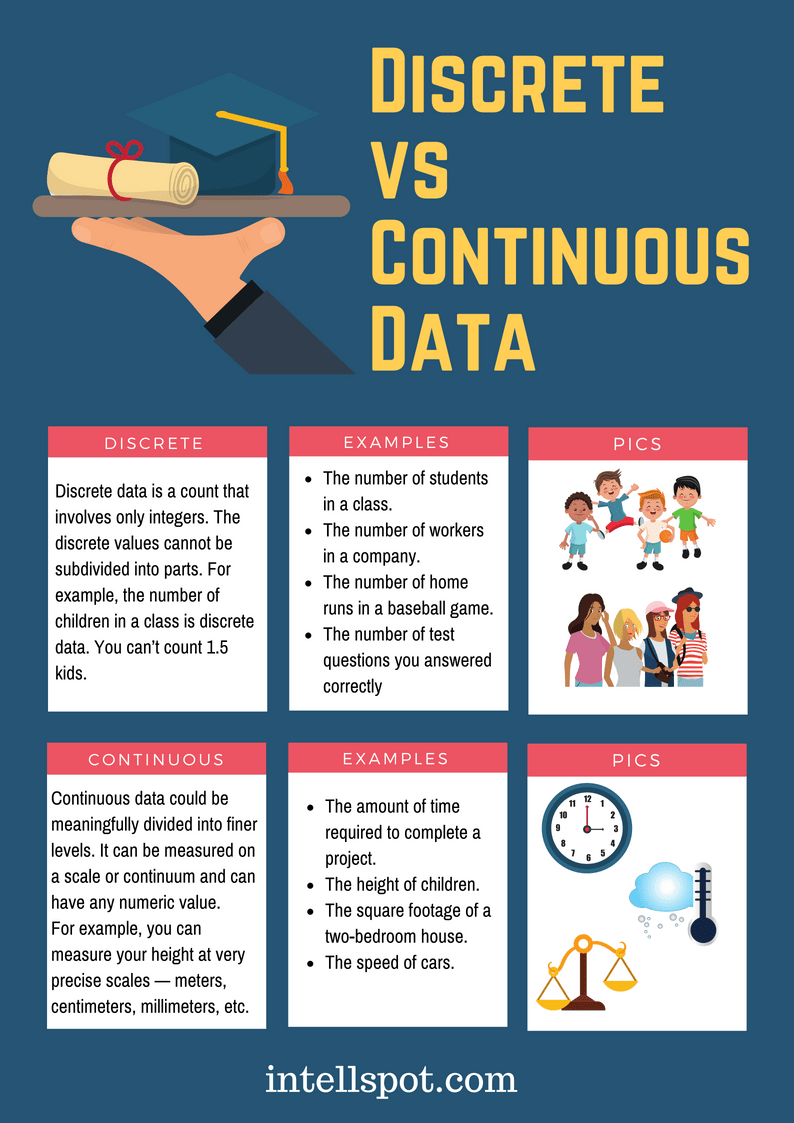# 6 Different Types of Data Every Data Scientist Must Know

If you know what are the different types of data (in statistics, maths, or data science), you are able to pick that data type that most closely matches your needs and purposes.

There aren’t only two or three types of data but a list of types you need to understand.

Why? Because the various types of classification of data allow you to correctly use data measurements and thus to correctly make conclusions about it.

On this page:

• The most common data types (with examples) every statistician and data scientist should know
• Infographics in PDFQualitative vs Quantitative Data

1. Quantitative data

Quantitative data can be expressed as a number or can be quantified. Simply put, quantitative data can be measured by numerical variables.

Quantitative data are easily amenable to statistical manipulation and can be represented by a wide variety of statistical types of graphs and chards such as line, bar graph, scatter plot, and etc.

Examples of quantitative data:

• Scores on tests and exams e.g. 85, 67, 90 and etc.
• The weight of a person or a subject.
• Your shoe size.
• The temperature in a room.

There are 2 general types of quantitative data: discrete data and continuous data. We will explain them later in this article.

2. Qualitative data

Qualitative data can’t be expressed as a number and can’t be measured. Qualitative data consist of words, pictures, and symbols, not numbers.

Qualitative data is also called categorical data.

Examples of qualitative data:

• Colors e.g. the color of the sea
• Your favorite holiday destination such as Hawaii, New Zealand and etc.
• Names as John, Patricia,…..
• Ethnicity such as American Indian, Asian, etc.

More you can see on our post qualitative vs quantitative data.

There are 2 general types of qualitative data: nominal data and ordinal data. We will explain them after a while.

Download the following infographic in PDFNominal vs Ordinal Data

3. Nominal data

Nominal data is used just for labeling variables, without any type of quantitative value. The name ‘nominal’ comes from the Latin word “nomen” which means ‘name’.

The nominal data just name a thing without applying it to an order. Actually, the nominal data could just be called “labels.”

Examples of Nominal Data:

• Gender (Women, Men)
• Hair color (Blonde, Brown, Brunette, Red, etc.)
• Marital status (Married, Single, Widowed)
• Ethnicity (Hispanic, Asian)

As you see from the examples there is no intrinsic ordering to the variables. Eye color is a nominal variable having a few categories (Blue, Green, Brown) and there is no way to order these categories from highest to lowest.

4. Ordinal data

Ordinal data shows where a number is in order. This is the crucial difference with nominal types of data.

Ordinal data is data which is placed into some kind of order by their position on a scale. Ordinal data may indicate superiority. However, you cannot do arithmetic with ordinal numbers because they only show sequence.

Ordinal variables are considered as “in between” qualitative and quantitative variables.

In other words, the ordinal data is a qualitative data for which the values are ordered.

In comparison with nominal data, the second one is a qualitative data for which the values cannot be placed in an ordered.

We can also assign numbers to ordinal data to show their relative position. But we can not do math with those numbers. For example: “first, second, third…etc.”

Examples of Ordinal Data:

• The first, second and third person in a competition.
• Letter grades: A, B, C, and etc.
• When a company asks a customer to rate the sales experience on a scale of 1-10.
• Economic status: low, medium and high.

Much more on the topic plus a quiz, you can learn in our post: nominal vs ordinal data.

Download the following infographic in PDFDiscrete vs Continuous Data

As we mentioned above discrete and continuous data are the two key types of quantitative data.

In statistics and data science, many decisions depend on whether the basic data is discrete or continuous.

5. Discrete data

Discrete data is a count that involves only integers. The discrete values cannot be subdivided into parts. For example, the number of children in a class is discrete data. You can count whole individuals. You can’t count 1.5 kids.

To put in other words, discrete data can take only certain values. The data variables cannot be divided into smaller parts.

It has a limited number of possible values e.g. days of the month.

Examples of discrete data:

• The number of students in a class.
• The number of workers in a company.
• The number of home runs in a baseball game.
• The number of test questions you answered correctly

6. Continuous data

Continuous data is information that could be meaningfully divided into finer levels. It can be measured on a scale or continuum and can have almost any numeric value.

For example, you can measure your height at very precise scales — meters, centimeters, millimeters and etc.

You can record continuous data at so many different measurements – width, temperature, time, and etc. This is where the key difference with discrete types of data lies.

The continuous variables can take any value between two numbers. For example, between 50 and 72 inches, there are literally millions of possible heights: 52.04762 inches, 69.948376 inches and etc.

A good great rule for defining if a data is continuous or discrete is that if the point of measurement can be reduced in half and still make sense, the data is continuous.

Examples of continuous data:

• The amount of time required to complete a project.
• The height of children.
• The square footage of a two-bedroom house.
• The speed of cars.

Much more on the topic you can see in our detailed post discrete vs continuous data: with a comparison chart.

Download the following infographic in PDFConclusion

All of the different types of data have a critical place in statistics and data science. Data types work great together to help organizations and businesses from all industries build successful data-driven decision-making process.

Working in the data management area and having a good range of data science skills involves a deep understanding of various types of data and when to apply them.